Publicité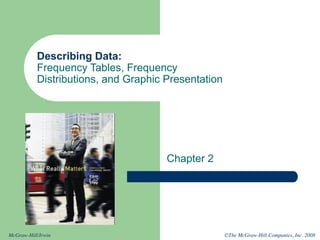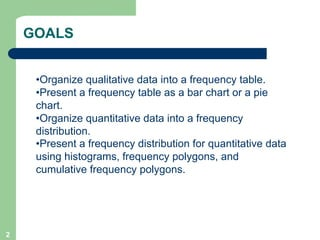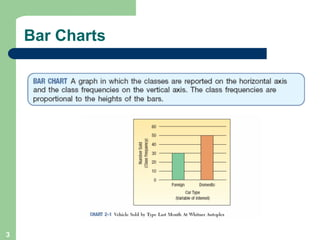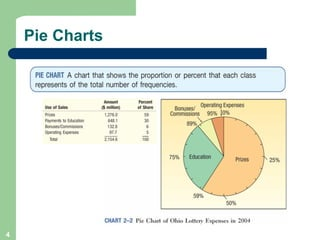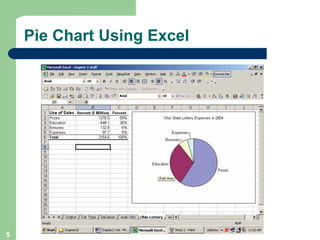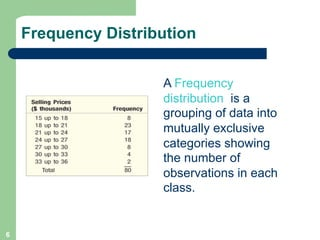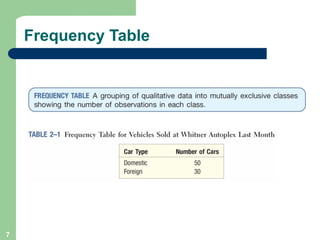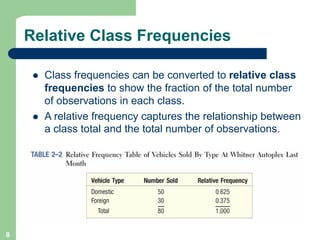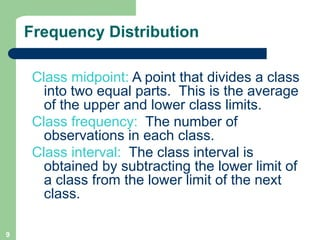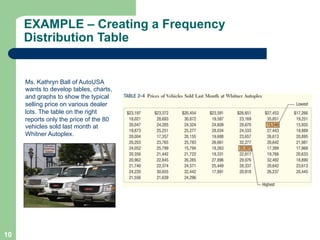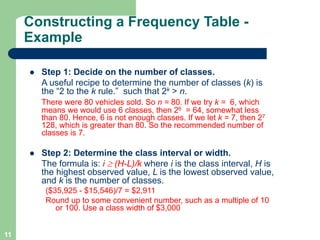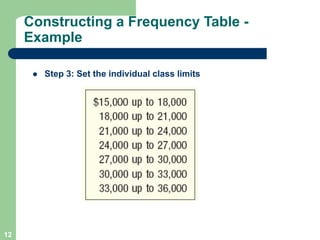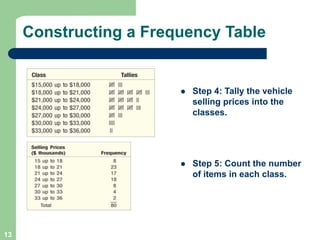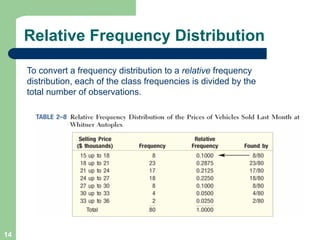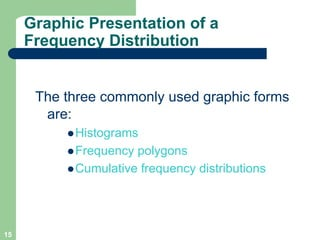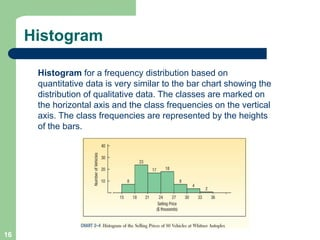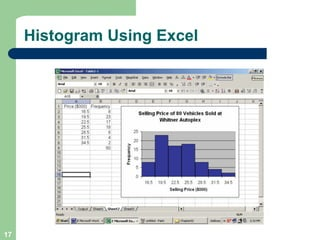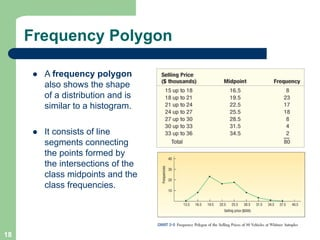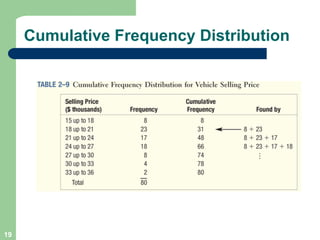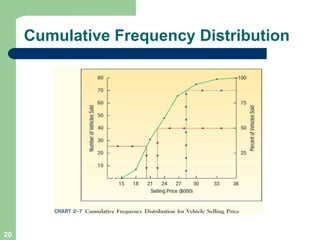1 sur 21
Publicité

### Chapter 02 (1).pdf

1. ©The McGraw-Hill Companies, Inc. 2008 McGraw-Hill/Irwin Describing Data: Frequency Tables, Frequency Distributions, and Graphic Presentation Chapter 2
2. 2 GOALS •Organize qualitative data into a frequency table. •Present a frequency table as a bar chart or a pie chart. •Organize quantitative data into a frequency distribution. •Present a frequency distribution for quantitative data using histograms, frequency polygons, and cumulative frequency polygons.
3. 3 Bar Charts
4. 4 Pie Charts
5. 5 Pie Chart Using Excel
6. 6 Frequency Distribution A Frequency distribution is a grouping of data into mutually exclusive categories showing the number of observations in each class.
7. 7 Frequency Table
8. 8 Relative Class Frequencies l Class frequencies can be converted to relative class frequencies to show the fraction of the total number of observations in each class. l A relative frequency captures the relationship between a class total and the total number of observations.
9. 9 Frequency Distribution Class midpoint: A point that divides a class into two equal parts. This is the average of the upper and lower class limits. Class frequency: The number of observations in each class. Class interval: The class interval is obtained by subtracting the lower limit of a class from the lower limit of the next class.
10. 10 EXAMPLE – Creating a Frequency Distribution Table Ms. Kathryn Ball of AutoUSA wants to develop tables, charts, and graphs to show the typical selling price on various dealer lots. The table on the right reports only the price of the 80 vehicles sold last month at Whitner Autoplex.
11. 11 Constructing a Frequency Table - Example l Step 1: Decide on the number of classes. A useful recipe to determine the number of classes (k) is the “2 to the k rule.” such that 2k > n. There were 80 vehicles sold. So n = 80. If we try k = 6, which means we would use 6 classes, then 26 = 64, somewhat less than 80. Hence, 6 is not enough classes. If we let k = 7, then 27 128, which is greater than 80. So the recommended number of classes is 7. l Step 2: Determine the class interval or width. The formula is: i ³ (H-L)/k where i is the class interval, H is the highest observed value, L is the lowest observed value, and k is the number of classes. (\$35,925 - \$15,546)/7 = \$2,911 Round up to some convenient number, such as a multiple of 10 or 100. Use a class width of \$3,000
12. 12 l Step 3: Set the individual class limits Constructing a Frequency Table - Example
13. 13 l Step 4: Tally the vehicle selling prices into the classes. l Step 5: Count the number of items in each class. Constructing a Frequency Table
14. 14 Relative Frequency Distribution To convert a frequency distribution to a relative frequency distribution, each of the class frequencies is divided by the total number of observations.
15. 15 Graphic Presentation of a Frequency Distribution The three commonly used graphic forms are: lHistograms lFrequency polygons lCumulative frequency distributions
16. 16 Histogram Histogram for a frequency distribution based on quantitative data is very similar to the bar chart showing the distribution of qualitative data. The classes are marked on the horizontal axis and the class frequencies on the vertical axis. The class frequencies are represented by the heights of the bars.
17. 17 Histogram Using Excel
18. 18 Frequency Polygon l A frequency polygon also shows the shape of a distribution and is similar to a histogram. l It consists of line segments connecting the points formed by the intersections of the class midpoints and the class frequencies.
19. 19 Cumulative Frequency Distribution
20. 20 Cumulative Frequency Distribution
21. 21 End of Chapter 2
Publicité